## Q-learning

Q-learning is an off-policy, model-free RL algorithm and its goal is to approximate the optimal action-value function $Q ^{\ast}$, which in turn gives us an optimal policy (by acting greedily wrt $Q ^{\ast}$). In other words, this algorithm provides agents with the capability of learning to act optimally by collecting experiences in a possibly unknown MDP, without requiring them to build a concrete model of their environment. The Q-learning algorithm is basically an intelligent adaptation of policy iteration, which was described in our last blog post. The core idea of policy iteration was to iteratively switch between policy improvement (greedy actions) and policy evaluation (calculate related Q-function). As mentioned before policy evaluation is not a trivial procedure, since - in theory - one would need to apply the Bellman operator infinitely often for every state-action pair. In “large” MDPs this strategy is basically infeasible.

Thus one needs to find ways to transfer those concepts into more applicable algorithms. In the case of Q-learning there is a difference between the evaluated policy and the behavior policy (off-policy method). This means that in every iteration step $k$ we still try to evaluate the greedy policy $\pi_k$ wrt the preceding Q-function $Q_{k-1}$ (target policy), but at the same time we will interact with the environment in terms of a slightly different policy $\bar{\pi}_k$. Furthermore, at every iteration step we wont fully evaluate the target policy $\pi_k$, but rather just apply the suitable Bellman optimality operator once for one state-action pair (asynchronous Dynammic Programming). Which state-action pair gets evaluated is fully determined by the experiences generated by the behavior policy $\bar{\pi}_k$. As a consequence, we can not expect that $Q_k = Q _{\pi_k}$, i.e. $Q _k$ is only an estimate of $Q _{\pi_k}$. Due to the fact that we don’t want to consider every state-action pair at every iteration step, the sequence of behavior policies $(\bar{\pi}_k) _ {k \in \mathbb{N}}$ has to guarantee that we at least visit every state-action pair every now and then. Otherwise some state-action pairs are ignored and it would be unrealistic to expect convergence of the algorithm.

In practice, the behavior policy $\bar{\pi} _k$ is often just the $\epsilon$ -greedy policy wrt $Q _{k-1}$ with $\epsilon \in [ 0,1 ], \epsilon « 1$, i.e. in state $s \in \mathcal{S}$ with probability $1 - \epsilon$ take a greedy action $a \in argmax _{a \in \mathcal{A}} Q _{k-1} (s,a)$ and with probability $\epsilon$ take a random action $a \in \mathcal{A}$. Considering again an infinite-horizon MDP with a finite state- and action-space, this $\epsilon$-greedy policy $\bar{\pi}_k$ obviously makes sure that every state-action pair is visited infinitely often.

To clarify the sketched Q-learning algorithm, let us write down a detailed version of the algorithm. In the following form of the algorithm we consider MDPs with terminal states. One sequence of experiences $<s_0,a_0,R_0,s_1,a_1,R_1,…,s_T>$, where $s_t \in \mathcal{S}, a_t \in \mathcal{A}, R_t = \mathcal{R} (s_t,a_t)$, $s_0$ an initial and $s_T$ a terminal state, is called an episode: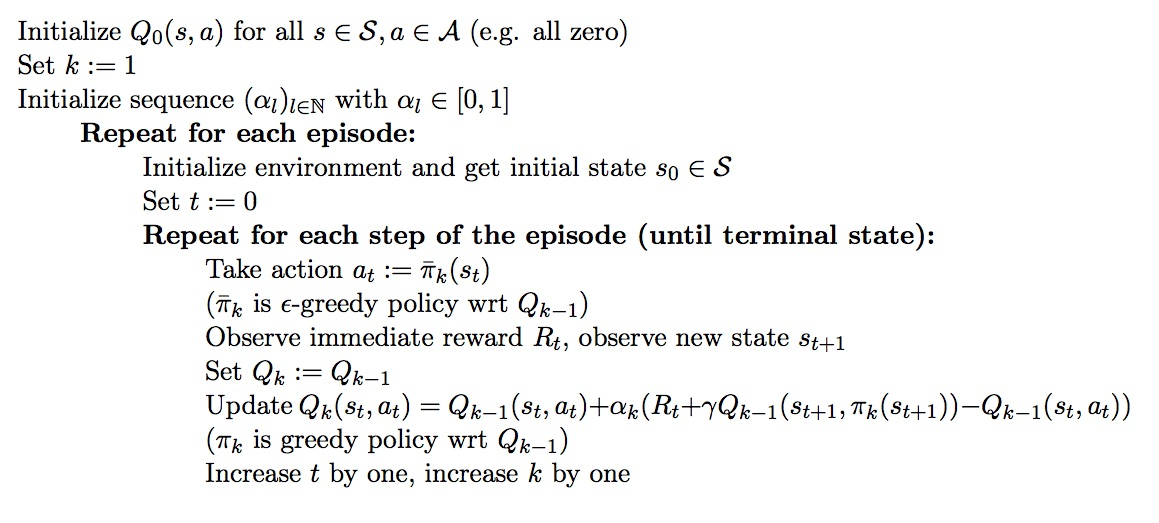A few little aspects of the algorithm still have to be discussed. Hopefully you remember that the Bellman Optimality operator applied to $Q_{k-1}$ at the tupel $<s_t,a_t>$ was originally defined by:

You might notice that my statement above, about applying the Bellman operator once in the Q-learning algorithm, is not fully true. Instead we update $Q_{k-1}$ only slightly in the direction of the operator by making use of a sequence $(\alpha_l) _{l \in \mathbb{N}}$. This is motivated by the fact that we learn about the Q-function while collecting experiences (here we experienced $<s _t,a _t,R _t,s _{t+1}>$) without having any knowledge about the transition dynamics and the other possible states $s’ \in \mathcal{S}$, where we could have ended up in after taking action $a_t$ in $s_t$. Considering the fact that we visit $<s_t,a_t>$ again and again over the life of the agent, the sequence $(\alpha) _{l \in \mathbb{N}}$ should help us to calculate an incremental mean of the value $R _t + \gamma \sum _{ s’ \in \mathcal{S} } \mathcal{P} (s _t,a _t,s’) Q _{k-1} (s’, \pi_k (s’))$, i.e. $\alpha_l$ has to decrease with increasing $l$.

Although Q-learning has now been explained in detail, it is not at all clear why this should actually converge to the desired optimal action-value function $Q ^{\ast}$. And to be honest, we can only guarantee convergence under certain additional assumptions. There are cases, e.g. if we use non-linear function approximators for $Q_k$, where Q-learning can diverge. However, even in those cases Q-learning might still yield good results in practice.

The inventor of Q-learning Chris Watkins provided a convergence proof under the following (comparable) conditions: As before, a infinite-horizon MDP with finite state- and action-spaces is given. Besides, the reward function $\mathcal{R}$ is bounded and the discount factor $\gamma < 1$. The sequence $(\alpha_l) _{l \in \mathbb{N}} \subseteq [ 0,1 ]$ is of the Robbins-Monro type, i.e. $\sum _{l \in \mathbb{N}} \alpha_l = \infty$ and $\sum _{l \in \mathbb{N}} \alpha_l^2 < \infty$ (e.g. $\alpha_l = 1/l$). Furthermore, we assume that the functions $Q_k$ are represented in a tabular manner (look-up table representation), i.e. no function approximation. Then $Q_k \rightarrow Q^{\ast}$ with probability $1$. The proof of Watkins is quite interesting (key point: construction of a special action-replay process ARP), but would certainly go beyond the scope of this blog post. The interested reader should just have a look at the “Q-learning” (Watkins, Dayan) paper from 1992.

In order to get a better feeling for Q-learning, we will now present a simple implementation of the algorithm with a tabular-representation of $Q_k$.

### Example

In this example Q-learning will be applied to the “Taxi-v2” environment of the OpenAI Gym. In the “Taxi-v2” environment the agent is a taxi driver. The goal is to pick up a customer and bring him to his desired location. In order to do this, one has to drive through a maze-like 2D-world.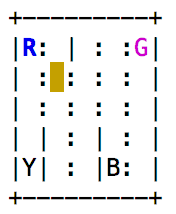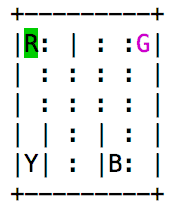Here, you see two different states of the environment. The letters R,G,Y,B are the possible pick-up and dropoff places. The blue color indicates the current location of the customer, and the pink color indicates his desired location. The agent’s taxi is this yellow square, but it turns green as soon as the agent has a passenger in the taxi. Overall there are 500 different possible states of the environment (i.e. $\mathcal{S} = <1,2,…,500>$), and in every state the agent has 6 different possible actions. He can try to go south ($0$), go north ($1$), go east ($2$), go west ($3$), pickup ($4$) and dropoff ($5$) - i.e. $\mathcal{A} = <0,…,5>$. This environment has terminal states; to be more precise, the game ends as soon as the passenger was dropped of at the right location. This success implies a reward of $+20$ for the agent. If the agent wants to drive through a wall, the action will simply don’t have any effect. Furthermore, if the agent tries to pickup or dropoff at a wrong location, it will experience an additional punishment (negative reward of $-10$). Besides, the agent constantly experiences small punishments ($-1$ per time step). These constant punishments shall motivate the agent to solve the problem at hand rather quickly.

Due to the fact that this environment only has $500 \times 6$ many state-action tupels, it makes sense to tackle this MDP with a Q-function that is represented by a matrix (tabular case). We will now implement the Q-algorithm as described above:

import gym
import numpy as np

env = gym.make("Taxi-v2") # create environment
Q = np.zeros(shape=(500, 6)) #rows = states, columns = actions

eps = 0.1 # epsilon value for behavior policy
gam = 1 # discount factor
EPISODE = 2000 # number of episodes during learning

for episode in range(EPISODE):
state = env.reset() # start new episode, save start-state
n = 1 # alpha sequence is 1/n with increasing n
done = False # boolean to indicate terminal state of environment
while done == False:
x = np.random.uniform(size=1)
if x <= eps:
action = np.random.randint(low=0, high=6)
else:
action = np.argmax(Q[state])

state_new, reward, done, _ = env.step(action) # execute action

Q[state, action] += 1/n*(reward + gam * np.max(Q[state_new])-Q[state,action])
n += 1
state = state_new


In most cases, it is already enough to play the game $2000$-times to find optimal policies. Although the $Q$-matrix probably hasn’t converged to the true optimal state-action values yet, it still results in an optimal policy when acting greedily wrt $Q$. Let us now test, whether the Q-algorithm was able to find an optimal policy. In this inference phase, we just try to play one episode with the help of our learned Q-function:

state = env.reset() # start new episode
env.render() # visualize the starting state
done = False
while done == False:
action = np.argmax(Q[state]) # act greedily
state, _ , done, _ = env.step(action)
env.render()


The above code yields the following terminal output: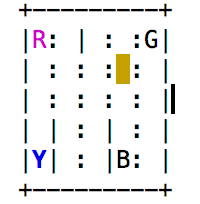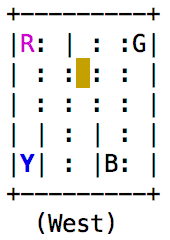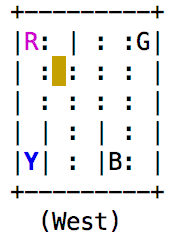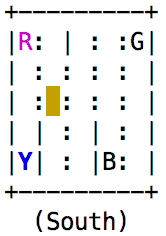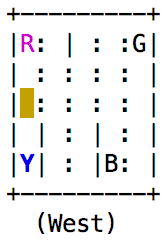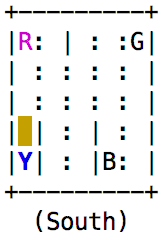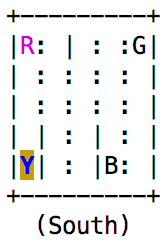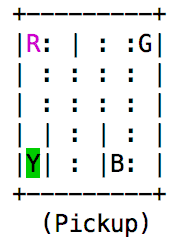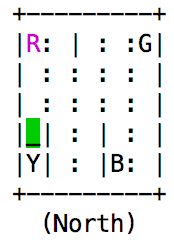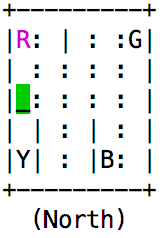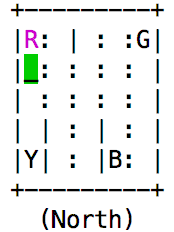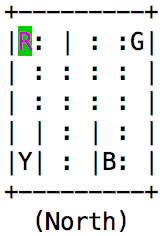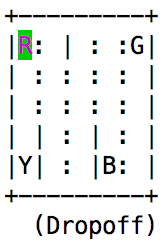In this game the agent certainly acted optimally! Thus the Q-learning algorithm really helped us to find an optimal policy.

In the next blog post we will finally introduce the Deep Q-Network (DQN), which is an impressive application of Q-learning. Here, we will use deep neural networks to represent $Q_k$ in every iteration step.

I hope you enjoyed this blog post!